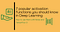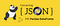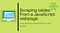# Why Rectified Linear Unit (ReLU) in Deep Learning and the best practice to use it with TensorFlow

## A practical introduction to ReLU with Keras and TensorFlow 2

The activation functions are at the very core of Deep Learning. They determine the output of a model, its accuracy, and computational efficiency. In some cases, activation functions have a major effect on the model’s ability to converge and the convergence speed.

In this article, you’ll learn why ReLU is used in Deep Learning and the best practice to use it with Keras and TensorFlow 2.

1. What is Rectified Linear Unit (ReLU)
2. Training a deep neural network using ReLU
3. Best practice to use ReLU with He initialization
4. Comparing to models with Sigmoid and…

# 7 popular activation functions you should know in Deep Learning and how to use them with Keras and TensorFlow 2

## A practical introduction to Sigmoid, Tanh, ReLU, Leaky ReLU, PReLU, ELU, and SELU7 popular activation functions in Deep Learning (Image by author using canva.com)

In artificial neural networks (ANNs), the activation function is a mathematical “gate” in between the input feeding the current neuron and its output going to the next layer .

The activation functions are at the very core of Deep Learning. They determine the output of a model, its accuracy, and computational efficiency. In some cases, activation functions have a major effect on the model’s ability to converge and the convergence speed.

In this article, you’ll learn the following most popular activation functions in Deep Learning and how to use them with Keras and TensorFlow 2.

1. Hyperbolic Tangent (Tanh)
2. Rectified Linear Unit…

# Building Custom Callbacks with Keras and TensorFlow 2

## A practical introduction to the custom callback

Callbacks are an important type of object in Keras and TensorFlow. They are designed to be able to monitor the model performance in metrics at certain points in the training run and perform some actions that might depend on those performances in metric values.

Keras has provided a number of built-in callbacks, for example, `EarlyStopping`, `CSVLogger`, `ModelCheckpoint`, `LearningRateScheduler` etc. Apart from these popular built-in callbacks, there is a base class called `Callback` which allows us to create our own callbacks and perform some custom actions. …

# How to convert JSON into a Pandas DataFrame

## Some of the most useful Pandas tricksconverting JSON into a Pandas DataFrame (Image by Author using canva.com)

Reading data is the first step in any data science project. Often, you’ll work with data in JSON format and run into problems at the very beginning. In this article, you’ll learn how to use the Pandas built-in functions `read_json()` and `json_normalize()` to deal with the following common problems:

1. Reading simple JSON from a URL
2. Flattening nested list from JSON object
3. Flattening nested list and dict from JSON object
4. Extracting a value from deeply nested JSON

Please check out Notebook for the source code.

# 1. Reading simple JSON from a local file

Let’s begin with a simple example.

`[  {    "id": "A001",    "name": "Tom",    "math": 60,    "physics": 66,    "chemistry": 61  },  {    "id": "A002",    "name": "James",    "math": 89,    "physics": 76,    "chemistry": 51  },  {    "id": "A003",    "name": "Jenny",    "math": 79,    "physics": 90,    "chemistry": 78  }…`

# Scraping tables from a JavaScript webpage using Selenium, BeautifulSoup, and Pandas

## A step by step tutorial for scraping tables from a JavaScript webpageScraping tables from a JavaScript webpage using Selenium, BeautifulSoup, and Pandas (Image by author using canva.com)

Web scraping is the process of collecting and parsing data from the web. The Python community has come up with some pretty powerful web scrapping tools. However, many modern websites are dynamic, in which the content is loaded and populated using client JavaScript. Therefore, some extra setups are required in order to scrape data from JavaScript webpages.

In this article, you’ll learn how to scrape tables from a JavaScript webpage using Selenium, BeautifulSoup, and Pandas.

1. Install libraries and Selenium web driver
2. Scrap tables using Selenium, BeautifulSoup, and Pandas

Please check out the source code from…

# All Pandas read_html() you should know for scraping data from HTML tables

Web scraping is the process of collecting and parsing data from the web. The Python community has come up with some pretty powerful web scrapping tools. Among them, Pandas `read_html()` is a quick and convenient way for scraping data from HTML tables.

In this article, you’ll learn Pandas `read_html()` to deal with the following common problems and should help you get started with web scraping.

1. Reading tables from a URL
2. Reading tables from a file
3. Parsing date columns with `parse_dates`
4. Explicitly typecast with `converters`
5. MultiIndex, header, and index column
6. Matching a table with `match`
7. Filtering tables with…

# All the Pandas shift() you should know for data analysis

## Some Pandas tricks to help you get started with data analysis

Suppose you encountered a situation where you need to push all rows in a DataFrame or require to use the previous row in a DataFrame. Maybe you want to calculate the difference in consecutive rows, Pandas `shift()` would be an ideal way to achieve these objectives.

In this article, we’ll be going through some examples of manipulating data using Pandas `shift()` function. We will focus on practical problems and should help you get started with data analysis.

1. Shifting time-series data with `freq`
2. A practical example: calculating the difference in consecutive rows
3. A practical example: calculating the 7 days difference for time-series…

# All the Pandas merge() you should know for combining datasets

## Some of the most useful Pandas tricks you should know

Pandas provides various built-in functions for easily combining datasets. Among them, `merge()` is a high-performance in-memory operation very similar to relational databases like SQL. You can use `merge()` any time when you want to do database-like join operations.

In this article, we’ll be going through some examples of combining datasets using Pandas `merge()` function. We will cover the following common usages and should help you get started with data combinations.

1. Specifying key columns using `on`
2. Merging using `left_on` and `right_on`
3. Various forms of joins: `inner`, `left`, `right` and `outer`
4. Using `validate` to avoid invalid…

# Pandas resample() tricks you should know for manipulating time-series data

## Some of the most useful Pandas tricks you should know

Time-series data is common in data science projects. Often, you may be interested in resampling your time-series data into the frequency that you want to analyze data or draw additional insights from data .

In this article, we’ll be going through some examples of resampling time-series data using Pandas `resample()` function. We will cover the following common problems and should help you get started with time-series data manipulation.

1. Downsampling with a custom base
2. Upsampling and filling values
3. A practical example

Please check out the notebook for the source code.

# 1. Downsampling and performing aggregation

Downsampling is to resample a time-series dataset to a wider time frame. For example, from minutes to hours, from days to years. The result will have a reduced number of rows and values can be aggregated with `mean()`, `min()`, `max()`, `sum()` etc. …

# Pandas concat() tricks you should know to speed up your data analysis

## Some of the most useful Pandas tricks you should know

Pandas provides various built-in functions for easily combining DataFrames. Among them, the `concat()` function seems fairly straightforward to use, but there are still many tricks you should know to speed up your data analysis.

In this article, you’ll learn Pandas `concat()` tricks to deal with the following common problems:

1. Avoiding duplicate indices
2. Adding a hierarchical index with `keys` and `names` options
3. Column matching and sorting

Please check out my Github repo for the source code.

# 1. Dealing with index and axis

`df1 = pd.DataFrame({    'name': ['A', 'B', 'C', 'D'],    'math': [60,89,82,70],    'physics': [66,95,83,66],    'chemistry': [61,91,77,70]…`